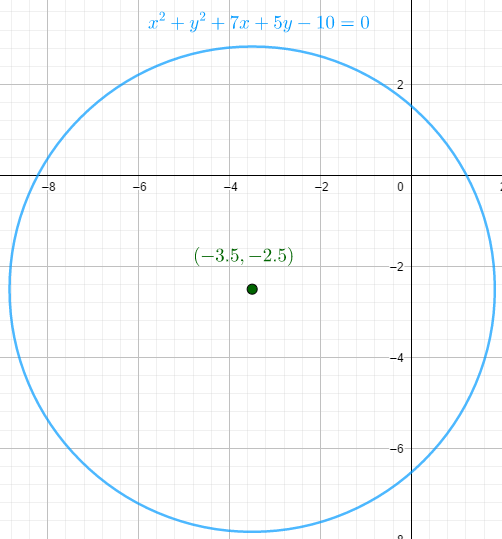### Math Notes

Subjects

#### Analytic Geometry Solutions

##### Topics || Problems

Plot the graph of the equation $$x^2 + y^2 +7x +5y-10=0$$.

To the plot the graph, determine the type of curve by writing the equation into its standard form.

$$x^2 + y^2 +7x +5y-10=0$$

$$x^2 +7x +y^2+5y=10$$

$$(x^2 +7x) + (y^2+5y)=10$$

$$(x^2 +7x + \frac{49}{4}) + (y^2+5y +\frac{25}{4})=10 + \frac{49}{4} + \frac{25}{4}$$, Completing the square

$$(x+\frac{7}{2})^2 +(y+\frac{5}{2})^2 = \frac{57}{2}$$

The equation represents a circle with center at $$(-3.5,-2.5)$$ and radius equal to $$5.339$$

Graph